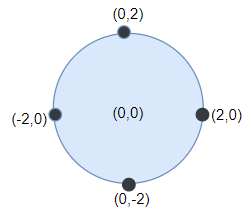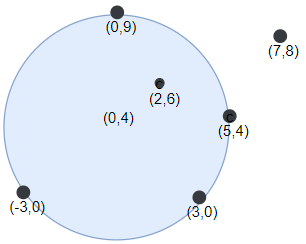# 圆形靶内的最大飞镖数量

## 1453. 圆形靶内的最大飞镖数量 (Hard)```输入：points = [[-2,0],[2,0],[0,2],[0,-2]], r = 2

``````输入：points = [[-3,0],[3,0],[2,6],[5,4],[0,9],[7,8]], r = 5

```输入：points = [[-2,0],[2,0],[0,2],[0,-2]], r = 1

```

```输入：points = [[1,2],[3,5],[1,-1],[2,3],[4,1],[1,3]], r = 2

```

• `1 <= points.length <= 100`
• `points[i].length == 2`
• `-10^4 <= points[i], points[i] <= 10^4`
• `1 <= r <= 5000`

[几何]